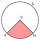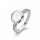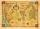# Area of the shape + direct relationship - math problems

#### Number of problems found: 31

• Circle segmentA quarter circle with radius 4 has the same content as a circle segment with radius 3. What is the magnitude of the center angle of the circle segment?
• Mr DumaMr Duma recently inherited a rectangular plot, part of the estate left by his late father. The plot with the following dimensions: Length=2x+1;Width=x-1. Determine the formula, in terms of x, that best describes the area of the rectangular plot. He has pl
• Jewelry boxThe bottom of Zeyda's jewelry box is a rectangle with the length of 5 3/8 inches and a width of 3 1/4 inches. What is the area of the bottom of the jewelry box?
• In theIn the national park, the ratio of the wooded area to grassland is 4: 1. The total area is 385km2. What area is wooded?
• Ratio of sides 2The ratio of the side lengths of one square to another is 1:2. Find the ratio of the area of the two squares.
• DivideDivide area of rectangles with dimensions 32m and 10m by the ratio 7: 9. What area corresponds to a smaller section?
• Map scaleThe rectangular plot has a scale of 1: 10000 area 3 cm2 on the map. What content does this plot have on a 1:5000 scale map?
• GardenThe rectangular garden has dimensions of 27 m and 30 m. Peter and Katka split it in a ratio of 4:5. How many square meters did Katkin measure part of the garden?
• The sidesThe sides of the rectangle are in a ratio of 3: 5 and its circumference measures 72 cm. Calculate: a) the size of both sides of the rectangle b) the area of the rectangle c) the length of the diagonals
• Isosceles trapezoidCalculate the content of an isosceles trapezoid whose bases are at ratio 5:3, the arm is 6cm long and it is 4cm high.
• Map scaleOn a 1:1000 scale map is a rectangular land of 4.2 cm and 5.8 cm. What is the area of this land in square meters?
• Rectangle 3-4-5The sides of the rectangle are in a ratio of 3:4. The length of the rectangle diagonal is 20 cm. Calculate the content of the rectangle.
• Rhombus and diagonalsThe rhombus area is 150 cm2, and the ratio of the diagonals is 3:4. Calculate the length of its height.
• The landThe land in the shape of a square has 9 ha. How big a page will the land have at the scale of 1: 5000?
• TrapeziumThe length of the base and the height size of the base of the trapezium is at ratio 5:3:2, the content area of the trapezium is 128 cm2. Calculate the length of the base and the height of the trapezoid.
• GlassAt the glass shop we have to cut 8 sheets of glass. Each was shaped a square with sides of 18 cm. We paid 44 CZK. How much is a 1 m2 of glass?
• TrapezoidArea of trapezoid is 135 cm2. Sides a, c and height h are in a ratio 6:4:3. How long are a,c and h? Make calculation...
• Painter 3Dad want to paint wall high 250 cm wide and 230 cm with wallpaper. How many meters must buy wallpaper if wallpaper width is 60 cm?
• Map scaleGarden has on plan on a scale of 1: 150 width 22 cm and length 35 cm. What is the real area of the garden?
• Ratio - rectangleThe rectangle has dimensions 6 cm and 9 cm. How many times increases area and its perimeter, when its dimensions increase in the ratio 5:3?

Do you have an exciting math question or word problem that you can't solve? Ask a question or post a math problem, and we can try to solve it.

We will send a solution to your e-mail address. Solved examples are also published here. Please enter the e-mail correctly and check whether you don't have a full mailbox.

Examples of area of plane shapes. Direct relationship - math problems.